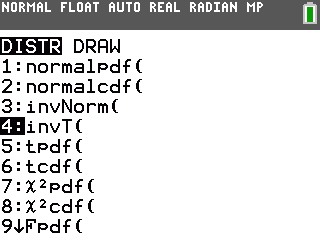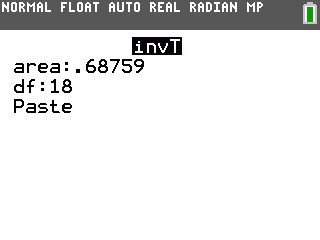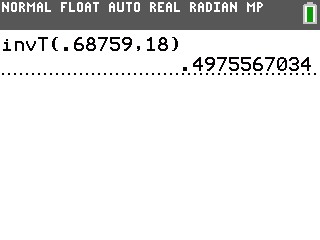# Knowledge Base

## Solution 34883: Calculating the Inverse Student-T (invT) Probability on the TI-84 Plus Family of Graphing Calculators.

### How can I calculate the inverse student-T probability on the TI-84 Plus family of graphing calculators?

The Inverse Student-T is usually used for determining probabilities of population means from sampled distributions when the standard deviation of the population is unknown. Specifically, t = (x-u)*Ö(n)/s with n-1 degrees of freedom and where x is the sampled mean, u is the population mean, s is the sample standard deviation, n is the sample size, and the population is Gaussian.

The command for this function is under the DISTR menu. Please refer to the instructions provided below given the below data.

Area = 0.68759
DF = 18

• Press [2ND][DISTR].
• Press  to select 4:InvT(.• Press [.]      [ENTER].
• Press   [ENTER].• Press [ENTER] to paste the command on the screen.
• Press [ENTER] to display the answer of 0.4975567034